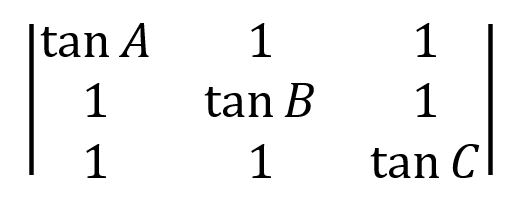# Everyday maths - 7(17^2)

Let’s solve and discuss maths questions together.
I will try to post one question every day with answer in the following 52*7+2 days, without any solution. Everyone is welcomed to post questions too, but this is not a homework thread -.-
(I do not set a specific topics or difficulty for the questions, but try to add interesting questions or solutions to here.)

Please post your full solution after 12 hours so we can leave time for the others to solve.

Day -2: 30th Dec 2022
Make sure no one is confused by this question to continue…

Solve
2(48-36)²+12+(-16÷8*2-9*8+4)÷(1/6)

-132

I feel disgusted by the “÷” sign ughSo you have faced it since kindergarten…

Day -1: 31st Dec 2022
Simplify:

I just prefer to use fractions instead of that disgusting sign…

fractions make things easier

Happy new year, countdown to 2024Week 1 Day 1:

Week 1 Day 2: 2nd Jan 2023
In triangle ABC, find the value of:This honestly kinda scared me for when i have to take college trig

lol just mix with algebra so maybe it looks quite scary

Week 1 Day 3: 3rd Jan 2023

In the kite PQRS as shown below, PQ=PS, QR=RS, given that RS = 8, ∠SRP=30°, ∠SPQ=120°, find the length of SP.Week 1 Day 4: 4th Jan 2023

Find the radius of incircle of the kite above.

nth day:

1+1=?

2

This is math but also code xP

someone submits 1478.14 item, 235.7 stack, 69.51 sc, and 5.25 dc
what is the value of `\$multiplier` once all of the equations have finished.

``````        \$multiplier = 1;

if (isset(\$_POST['item']) && is_numeric(\$_POST['item'])) {
\$multiplier = \$multiplier + \$_POST['item'];
}
if (isset(\$_POST['stack']) && is_numeric(\$_POST['stack'])) {
\$multiplier = \$multiplier + (\$_POST['stack'] * 64);
}
if (isset(\$_POST['sc']) && is_numeric(\$_POST['sc'])) {
\$multiplier = \$multiplier + (\$_POST['sc'] * 1728);
}
if (isset(\$_POST['dc']) && is_numeric(\$_POST['dc'])) {
\$multiplier = \$multiplier + (\$_POST['dc'] * 3456);
}

if (\$multiplier !== 1) {
\$multiplier = \$multiplier - 1;
}
\$multiplier = round(\$multiplier, 0);
\$_SESSION['multiplier'] = \$multiplier;
``````

If a ball, that is 6 meters above the ground, is thrown perfectly horizontally to the right, how long would it take to reach the ground? (Assume gravity is 9.81m/s)

and also, how far, horizontally, would the ball travel after reaching the ground?

USE THE METRIC SYSTEM PEOPLE

cuz nobody likes the Imperial/English System aside from the Americans

t ≈ 0.35 seconds
x ≈ 0.84 meters

Well I was using my free time to… play in server. Is it good or bad >.>
I’ll come back with 21 questions soon.

And, wait, maths with physics and codes? I’m surprised XD

Happy Rabbit and Cat Year!

I still can’t memorise most of the Metric equivalent of Imperial System, even sometimes we still use it hereCm = inch*2.54

I have it memorized

there’s also 15 Celsius = 59 Fahrenheit (these are room temperature or Standard Sea Level conditions)

1kg = 2.2 lbm (approx)

1BTU = 778 lbf ft = 1055 J

and many fricking more that makes it hard

imagine if the Americans also have their own unit of time… ugh

mm/dd/yyyy :pikalul: